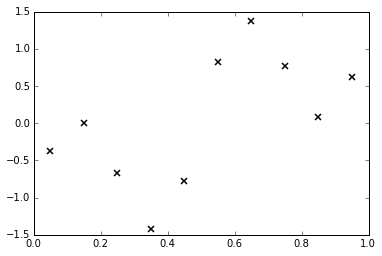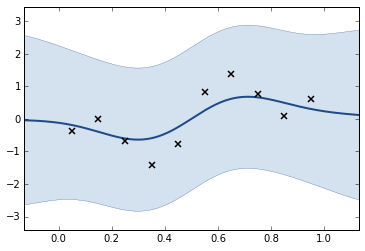# Gaussian Process Regression in GPy¶

## Gaussian Process Winter School, Genova, Italy¶

### Neil D. Lawrence and Nicolas Durrande¶

In the introduction to GPy you saw how it was possible to build covariance functions with the GPy software. The covariance function contains the assumptions about the data in it. In the Gaussian process, the covariance funciton encodes the model. However, to make predictions, you need to combine the model with data.

If the data we are given, $\mathbf{y}$ is real valued, then the problem is known as a regression problem. If a Gaussian noise model is used, then this is known as Gaussian process regression.

In :
%matplotlib inline
import numpy as np
import pods
import pylab as plt
import GPy
from IPython.display import display

/Users/neil/Library/Enthought/Canopy_64bit/User/lib/python2.7/site-packages/pytz/__init__.py:29: UserWarning: Module pods was already imported from /Users/neil/sods/ods/pods/__init__.pyc, but /Users/neil/Library/Enthought/Canopy_64bit/User/lib/python2.7/site-packages is being added to sys.path
from pkg_resources import resource_stream


We will now combine the Gaussian process prior with some data to form a GP regression model with GPy. We will generate data from the function $$f(x) = − \cos(\pi x ) + \sin(4\pi x )$$ over $[0, 1]$, adding some noise to give $y(x) = f(x) + \epsilon$, with the noise being Gaussian distributed, $\epsilon \sim \mathcal{N}(0, 0.01)$.

In :
X = np.linspace(0.05,0.95,10)[:,None]
Y = -np.cos(np.pi*X) + np.sin(4*np.pi*X) + np.random.normal(loc=0.0, scale=0.1, size=(10,1))
plt.figure()
plt.plot(X,Y,'kx',mew=1.5)

Out:
[<matplotlib.lines.Line2D at 0x10ee24150>]A GP regression model based on an exponentiated quadratic covariance function can be defined by first defining a covariance function,

In :
k = GPy.kern.RBF(input_dim=1, variance=1., lengthscale=0.2)


And then combining it with the data to form a Gaussian process model,

In :
model = GPy.models.GPRegression(X,Y,k)


Just as for the covariance function object, we can find out about the model using the command display(m).

In :
display(model)


Model: GP regression
Log-likelihood: -13.3414898005
Number of Parameters: 3

GP_regression. Value Constraint Prior Tied to
rbf.variance 1.0 +ve
rbf.lengthscale 0.2 +ve
Gaussian_noise.variance 1.0 +ve

Note that by default the model includes some observation noise with variance 1. We can see the posterior mean prediction and visualize the marginal posterior variances using

model.plot()

In :
_ = model.plot()The actual predictions of the model for a set of points Xstar (an $m \times p$ array) can be computed using

Ystar, Vstar, up95, lo95 = model.predict(Xstar)


### Exercise 1¶

What do you think about this first fit? Does the prior given by the GP seem to be appropriate?

### Exercise 2¶

The parameters of the models can be modified using the parameter name, for example

model.Gaussian_noise.variance = 0.001


Change the values of the parameters to obtain a better fit.

In :
# Exercise 2 answer here


As we saw when we introduced GPy and covariance functions, random sample paths from the conditional GP can be obtained using

np.random.multivariate_normal(mu[:,0],C)


Now you can sample paths from the posterior process by first obtaining the mean and covariance of the posterior, mu and C. These can be obtained from the predict method,

mu, C, up95, lo95 = model.predict(Xp,full_cov=True)


### Exercise 3¶

Obtain 10 samples from the posterior sample and plot them alongside the data below.

In :
# Exercise 3
`